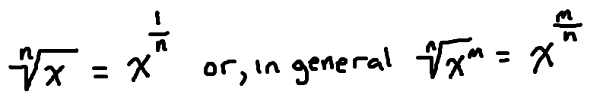# The power rule for integrals

The power rule for integrals allows us to find the indefinite (and later the definite) integrals of a variety of functions like polynomials, functions involving roots, and even some rational functions. If you can write it with an exponents, you probably can apply the power rule.To apply the rule, simply take the exponent and add 1. Then, divide by that same value. Finally, don’t forget to add the constant C.

## Integrals of polynomials

Finding the integral of a polynomial involves applying the power rule, along with some other properties of integrals. Take a look at the example to see how.

### Example

Find: $$\displaystyle\int 2x^3 + 4x^2 \text{ dx}$$

### Solution

We will write out every step here so that you can see the process. After some practice, you will probably just write the answer down immediately.

First, remember that integrals can be broken up over addition/subtraction and multiplication by constants. Therefore:

\begin{align} \displaystyle\int 2x^3 + 4x^2 \text{ dx} &= \displaystyle\int 2x^3\text{ dx} + \displaystyle\int 4x^2 \text{ dx}\\ &= 2\displaystyle\int x^3\text{ dx} + 4\displaystyle\int x^2 \text{ dx}\end{align}

Now apply the power rule by adding 1 to each exponent, and then dividing by the same number. When you do this, the integral symbols are dropped since you have “taken the integral”.

$$2\displaystyle\int x^3\text{ dx} + 4\displaystyle\int x^2 \text{ dx} = 2\left(\dfrac{x^{3+1}}{3+1}\right) + 4\left(\dfrac{x^{2+1}}{2+1}\right) + C$$

\begin{align} &=2\left(\dfrac{x^{3+1}}{3+1}\right) + 4\left(\dfrac{x^{2+1}}{2+1}\right) + C\\ =& 2\left(\dfrac{x^{4}}{4}\right) + 4\left(\dfrac{x^{3}}{3}\right) + C\\ & = \bbox[border: 1px solid black; padding: 2px]{\dfrac{x^4}{2} + \dfrac{4x^3}{3} + C}\end{align}

Now, let’s look at how this kind of integral would be with skipping some of the more straightforward steps.

### Example

Find: $$\displaystyle\int -3x^2 + x – 5 \text{ dx}$$

### Solution

This one is a little different. We have an $$x$$ by itself and a constant. For the $$x$$ by itself, remember that the exponent is 1. For the constant, remember that the integral of a constant is just the constant multiplied by the variable. For example, the integral of 2 with respect to $$x$$ is $$2x$$.

Applying the power rule:

$$\displaystyle\int -3x^2 + x – 5 \text{ dx} = -3\left(\dfrac{x^3}{3}\right) + \dfrac{x^2}{2} – 5x + C$$

$$= \bbox[border: 1px solid black; padding: 2px]{-x^3 + \dfrac{x^2}{2} – 5x + C}$$

## Integrals of radical functions (functions with roots)

In order to apply this rule to this type of function, you must remember one very important idea from algebra.So, if we can write the function using exponents then we can likely apply the power rule.

### Example

Find: $$\displaystyle\int \sqrt{x} + 4 \text{ dx}$$

Before applying any calculus, you can rewrite the integral using the rule above. This way, we have exponents and can apply the power rule.

$$\displaystyle\int \sqrt{x} + 4 \text{ dx} = \displaystyle\int {x}^{\frac{1}{2}} + 4 \text{ dx}$$

Now, applying the power rule (and the rule for integrating constants):

$$\displaystyle\int {x}^{\frac{1}{2}} + 4 \text{ dx} = \dfrac{x^{\frac{1}{2}+1}}{\frac{1}{2}+1} + 4x + C$$

Simplify to get the final answer:

\begin{align} &=\dfrac{x^{\frac{3}{2}}}{\frac{3}{2}} + 4x + C\\ &= \bbox[border: 1px solid black; padding: 2px]{\dfrac{2}{3}x^{\frac{3}{2}} + 4x + C}\end{align}

Usually, the final answer can be written using exponents like we did here or with roots. Your teacher or professor may have a preference, so make sure to ask!

Let’s work with one that is a little more messy with the fractions. As you will see, no matter how many fractions you are dealing with, the approach will stay the same.

### Example

Find: $$\displaystyle\int \dfrac{1}{2}\sqrt{x} + 5\sqrt{x^3} \text{ dx}$$

### Solution

Rewrite using algebra before you apply calculus rules so that you can use the power rule.

$$\displaystyle\int \dfrac{1}{2}\sqrt{x} + 5\sqrt{x^3} \text{ dx}= \displaystyle\int \dfrac{1}{2}x^{\frac{1}{3}} + 5x^{\frac{3}{4}} \text{ dx}$$

Apply the power rule:

$$\displaystyle\int \dfrac{1}{2}x^{\frac{1}{3}} + 5x^{\frac{3}{4}} \text{ dx} = \dfrac{1}{2}\left(\dfrac{x^{\frac{1}{3}+1}}{\frac{1}{3}+1}\right) + 5\left(\dfrac{x^{\frac{3}{4}+1}}{\frac{3}{4}+1}\right) +C$$

Simplify to get the final answer:

\begin{align} &= \dfrac{1}{2}\left(\dfrac{x^{\frac{4}{3}}}{\frac{4}{3}}\right) + 5\left(\dfrac{x^{\frac{7}{4}}}{\frac{7}{4}}\right) +C\\ &= \dfrac{1}{2}\left(\dfrac{3}{4}{x^{\frac{4}{3}}}\right) + 5\left(\dfrac{4}{7}x^{\frac{7}{4}}\right) +C\\ &= \bbox[border: 1px solid black; padding: 2px]{\dfrac{3}{8}x^{\frac{4}{3}} + \dfrac{20}{7}x^{\frac{7}{4}} +C}\end{align}

Did you notice that most of the work was with algebra? This is true of most calculus problems. The calculus part is straightforward while the algebra requires you to be very careful and makes up most of the problem.

## Integrals with negative exponents

One more old algebra rule will let us use the power rule to find even more integrals.Let’s see how we can apply it!

### Example

Find: $$\displaystyle\int \dfrac{3}{x^5} – \dfrac{1}{4x^2} \text{ dx}$$

Use the rule above and rewrite this integral with exponents. Pay special attention to what terms the exponent applies to.

$$\displaystyle\int \dfrac{3}{x^5} – \dfrac{1}{4x^2} \text{ dx} = \displaystyle\int 3x^{-5} – \dfrac{1}{4}x^{-2} \text{ dx}$$

Apply the power rule:

$$\displaystyle\int 3x^{-5} – \dfrac{1}{4}x^{-2} \text{ dx} = 3\left(\dfrac{x^{-5+1}}{-5+1}\right) – \dfrac{1}{4}\left(\dfrac{x^{-2+1}}{-2+1}\right) + C$$

Simplify to get the final answer:

\begin{align} &= 3\left(\dfrac{x^{-4}}{-4}\right) – \dfrac{1}{4}\left(\dfrac{x^{-1}}{-1}\right) + C\\ &= -\dfrac{3}{4}x^{-4} + \dfrac{1}{4}x^{-1} + C\\ &= -\dfrac{3}{4}\left(\dfrac{1}{x^4}\right) + \dfrac{1}{4}\left(\dfrac{1}{x}\right) + C\\ &= \bbox[border: 1px solid black; padding: 2px]{-\dfrac{3}{4x^4} + \dfrac{1}{4x} + C}\end{align}

Note that this only works when the exponent is not –1. If you tried to apply the power rule here, you would end up dividing by zero. There is a different rule for dealing with functions like $$\dfrac{1}{x}$$.